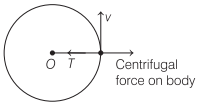# Centripetal Force and Centrifugal Force | Definition, Examples, Formulas – Motion in a Plane

Centripetal Force Definition:
It is that force which comes in to play when a body moves on a circular path. It is directed along radius of the circle towards its centre.

## Centripetal Force and Centrifugal Force | Definition, Examples, Formulas – Motion in a Plane

We are giving a detailed and clear sheet on all Physics Notes that are very useful to understand the Basic Physics Concepts.

Centripetal Force Formula:
Centripetal force, F = $$\frac{m v^{2}}{r}$$ = mrω²
where, m = mass of the body,
v = linear velocity,
ω = angular velocity and r = radius.
Work done by the centripetal force is zero because the centripetal force and displacement are at right angles to each other.

Examples of some incidents and the cause of centripetal force involved.

 Incidents Force Providing Centripetal Force Orbital motion of planets. Gravitational force between planet and sun. Orbital motion of electron. Electrostatic force between electron and nucleus. Turning of vehicles at turn. Frictional force acting between tyres of vehicle and road. Motion of a stone in circular path, tied with a string. Tension in the string.

Centrifugal Force Definition:
It is defined as the radially directed outward force acting on a body in circular motion as observed by the person moving with the body. It is equal in magnitude but opposite in direction to centripetal force.Centrifugal force does not act on the body in an inertial frame but arises as pseudo forces in non-inertial frames.

Motion in a Plane (Projectile and Circular Motion):
In this chapter or under this topic, we are going to come across the motion of the object when it is thrown from one end to another end. This practice is said to be projection. Also, when an object is moved in a circular motion, then the equation of the motion is derived here. We will learn here about centripetal force and centripetal acceleration in detail with formulas. Also learn the force applied in everyday life motion of the particle in a vertical circle.